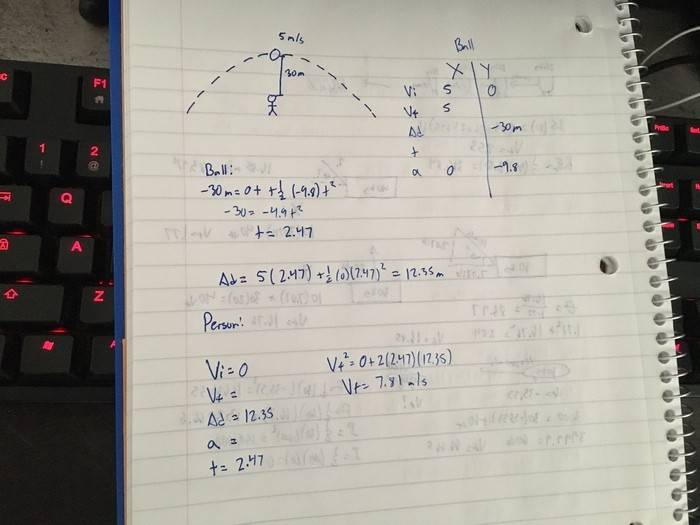# Algebra Kinematics: Ball above head, how fast must travel to

## Homework Statement

Hello there physicsforums,

I have a problem that I'd like feedback on as I'm not sure if I had solved it or approached it correctly.

A ball that is traveling 5m/s is 30 meters above a person who is standing still. How fast must the person travel in order to catch the ball?

To minimize confusion and misinterpretation I've decided to upload a visual of the problem, as well as my work involved:

http://imgur.com/m1tGOIA## Homework Equations

(Final velocity)^2 = (Initial velocity)^2 + 2 (acceleration)(change of distance)
(change of distance) = (Initial velocity)(time) + .5 (acceleration)(time)^2

## The Attempt at a Solution

My logic:

My first approach was to find the time it would take the ball to get from the top of its trajectory to the person.

Once I found the time, I used that time to calculate how far the ball would have traveled on the x axis.

Knowing how far the ball traveled in the x axis and how long it took, I then found the speed at which the person standing still would need to travel in order to cover that amount of distance in the certain amount of time.

I'm not sure if this is correct or not, but this was the only logical approach I could come up with.

Last edited:

gneill
Mentor
Hi Notaphysicsmajor,

Welcome to Physics Forums!

Your logic is sound, if a bit overkill for the problem. Consider: what is the x-velocity of the ball? Does it ever change during its flight?

In your image you write an equation: ##V_f^2 = 0 + 2(2.47)(12.35)##. It seems to me from your other work there that the 2.47 is a time value which seems strange. Is that the case? What's the original kinematic equation in symbols?

Excellent observation my dear Watson!

Thank you for catching that, yes you are correct.

The equation is (Final velocity)^2 = (Initial velocity)^2 + 2(acceleration)(distance), and I had input time in the value for acceleration.

Correct me if I'm wrong, I should have used the formula (change of distance) = (Initial velocity)(time) + .5(acceleration)(time)^2 to find acceleration.

With acceleration now known, I can use (Final velocity) = (Initial velocity) + (acceleration)(time)

After a quick computation here is what I was able to come up with:

(change of distance) = (Initial velocity)(time) + .5(acceleration)(time)^2
Δd = V° + .5(a)(t)^2
12.35 = 0(2.47) + .5(a)(2.47)^2
Acceleration = 4.05

(Final velocity) = (Initial velocity) + (acceleration)(time)
Vƒ = V° + (a)(t)
Vƒ = 0 + (4.05)(2.47)
Vƒ = 10

gneill
Mentor
The question only asks, "How fast must the person travel in order to catch the ball?". If you assume constant speed for "how fast" then he only needs to stay under the ball...

How fast must the person travel in order to catch the ball?
I thought the wording of this question was poor. What came to mind for me was that the person would be moving with a constant velocity. You have assumed that he would be accelerating with a constant velocity (EDIT: I mean constant acceleration). You may be right since it was stated that the person was originally "standing still".